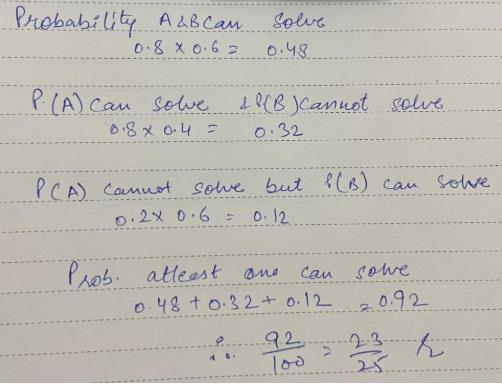Courses

# Test: Independent Events

## 10 Questions MCQ Test Mathematics (Maths) Class 12 | Test: Independent Events

Description
This mock test of Test: Independent Events for JEE helps you for every JEE entrance exam. This contains 10 Multiple Choice Questions for JEE Test: Independent Events (mcq) to study with solutions a complete question bank. The solved questions answers in this Test: Independent Events quiz give you a good mix of easy questions and tough questions. JEE students definitely take this Test: Independent Events exercise for a better result in the exam. You can find other Test: Independent Events extra questions, long questions & short questions for JEE on EduRev as well by searching above.
QUESTION: 1

Solution:
QUESTION: 2

Solution:
QUESTION: 3

### What is the probability of picking a spade from a normal pack of cards and rolling an odd number on a die?​

Solution:
QUESTION: 4

If A and B are two independent events, then

Solution:
QUESTION: 5

Two parts A and B of a machine is manufactured by a firm. Out of 100 A’s 12 are likely to be defective and Out of 100 B’s 8 are likely to be defective. The probability that a machine manufactured by the firm is free from any defect is:​

Solution:
QUESTION: 6

A and B are two independent events. The probability that both A and B occur is 1/6 and the probability that neither of them occurs is 1/3. The probability of occurrence of A is.​

Solution:
QUESTION: 7

A and B are two independent events. The probability that both A and B occur is 1/6 and the probability that neither of them occurs is 1/3. The probability of occurrence of A is.​

Solution:
QUESTION: 8

Ashmit can solve 80% of the problem given in a book and Amisha can solve 70%. What is the probability that at least one of them will solve a problem selected at random from the book?

Solution:QUESTION: 9

If A and B are independent events, such that P(A ∪ B)= 0.7, P(B) = 0.5, then P(A) = ……​

Solution:
QUESTION: 10

A student can solve 70% problems of a book and second student solve 50% problem of same book. Find the probability that at least one of them will solve a selected problem from this book.​

Solution: